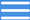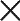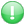MENUGet premium membership and access questions with answers, video lessons as well as revision papers.

# Grade 7 Mathematics video lessons and lectures

15 video courses

•  Grade 7 Data Handling and Probability Online Lessons

Grade 7 Data Handling and Probability Online Lessons. Areas Covered: 1. Meaning and collection of data 2. Representing data using a frequency distribution table 3. Choosing suitable scale for graphs 4. Representing data using pictographs and bar graphs 5. Interpreting bar graphs 6....

20 Video Lessons

•  Grade 7 Online Lessons on Decimals

Grade 7 Online Lessons on Decimals Areas Covered: 1. Place value of digits in decimals 2. Total value of digits in decimals 3. Multiplication and division of decimal numbers by whole numbers 4. Multiplication and division of decimal numbers by decimal numbers

30 Video Lessons

•  Grade 7 Mathematics Online Lessons on Fractions

Grade 7 Mathematics Online Lessons on Fractions Topic: Numbers Sub Topics: Fractions 1. Comparing fractions 2. Addition, subtraction, multiplication and reciprocal of fractions 3. Division of fractions by whole numbers 4. Division of whole numbers by fractions 5. Identifying and creating...

45 Video Lessons

•  Grade 7 Online Lessons in Factors

Grade 7 Online Lessons in Factors Topic: Numbers Sub-Topic: Factors 1. Divisibility tests of 2, 3, 4, 5, 6, 7, 8, 9, 10 and 11 2. Composite numbers 3. Greatest common divisor 4. Least common multiple 5. Real-life applications of GCD and LCM

25 Video Lessons

•  Grade 7 Online Lessons on Whole Numbers

GRADE 7 TOPIC: NUMBERS SUB TOPIC: WHOLE NUMBERS 1. Place value 2. Total value 3. Reading and writing numbers using cards and number charts 4. Reading and writing numbers in words 5. Rounding off numbers to the nearest million, tens of million, hundreds of million 6. Even and odd numbers 7....

57 Video Lessons

•  Grade 7 Mathematics Lessons on Measurement: Volume and Capacity

Grade 7 Mathematics Lessons on Measurement: Volume and Capacity 1. Conversion of cubic centimetres to cubic metres 2. Conversion of cubic metres to cubic centimetres 3. Volume of a cube, cuboid and cylinder 4. Relationship between cubic centimetres, cubic metres and litres 5. Relating volume to...

27 Video Lessons

•  Grade 7 Mathematics Online Lessons on Geometry: Angles

Grade 7 Mathematics Online Lessons on Geometry: Angles Areas Covered: 1. Angles on a straight line 2. Angles at a point 3. Alternate angles 4. Corresponding angles 5. Co-interior angles 6. Angles in a parallelogram 7. Angle properties of polygons 8. Interior and exterior angles of a...

25 Video Lessons

•  Grade 7 Online Videos on Measurements: Pythagorean Relationship

Grade 7 Online Videos on Measurements: Pythagorean Relationship 1. Sides of a right-angled triangle 2. Pythagorean relationship 3. Application of the pythagorean relationship

5 Video Lessons

•  Grade 7 Mathematics Online Lessons on Measurement: Length

Grade 7 Mathematics Online Lessons on Measurement: Length 1. Conversion of units of length 2. Addition, subtraction, division and multiplication of length 3. Perimeter of plane figures 4. Circumference of circles

30 Video Lessons

•  Grade 7 Mathematics Online Lessons of Measurements: Area

Grade 7 Mathematics Online Lessons of Measurements: Area Areas covered: 1. Acre and hectare as units of measuring area 2. Area of a rectangle, parallelogram, rhombus, trapezium, circle, borders and combined shapes

30 Video Lessons

•  Grade 7 Mathematics online lessons on squares and square roots

Grade 7 Mathematics online lessons on squares and square roots 1. Squares of whole numbers, fractions and decimals 2. Square roots of whole numbers, fractions and decimals

26 Video Lessons

Grade 7 Mathematics Online Lessons on Algebra: Questions and Answers Areas Covered: 1. Forming and simplifying algebraic expressions 2. Forming and simplifying linear equations 3. Solving linear equations 4. Application of linear equations 5. Forming simple linear inequalities 6. Illustrating...

60 Video Lessons

•  Grade 7 Online Video Lessons on Measurement: Temperature

Grade 7 Online Video Lessons on Measurement: Temperature Areas Covered: 1. Temperature conditions 2. Comparing temperature 3. Measuring temperature 4. Conversion of temperature from degrees Celsius to Kelvin 5. Conversion of temperature from Kelvin to degrees Celsius 6. Working out...

10 Video Lessons

•  Grade 7 Video Lessons on Measurement: Time, Distance and Speed

Grade 7 Video Lessons on Time, Distance and Speed. Areas Covered: 1. Units of measuring time 2. Conversion of units of measuring distance 3. Conversion of units of measuring time 4. Calculation of speed in metres per second and kilometres per hour 5. Conversion of speed from km/h to m/s and...

21 Video Lessons

•  Grade 7 Mathematics Online Lessons on Money

Grade 7 Mathematics Online Lessons on Money: Areas Covered 1. Profit and loss 2. Percentage profit and loss 3. Discount 4. Percentage discount 5. Commission 6. Percentage commission 7. Bills at home 8. Preparing bills 9. Inland postal charges 10. International surface mail postal...

27 Video Lessons